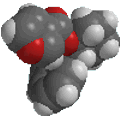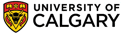Chapter 17: Aldehydes and Ketones. Nucleophilic Addition to C=OSpectroscopic Analysis of Aldehydes

• IR  - presence of , CH=O
 Absorbance (cm-1) Interpretation 1710 -1750 C=O stretch ~2720 C-H stretch of aldehydes ~2820 C-H stretch of aldehydes
• 1H NMR - the CH=O unit of aldehydes is the most easily recognised.
 Resonance (ppm) Interpretation 9.0 -10.0 CH=O (deshielding due to O and C=C) ~2.4 CH2C=O ~2.0 CH3C=O

-coupling (J, Hz) between protons in HC-CH=O is much smaller than HC-CH=C.

• 13C NMR

• C=O typically 190-220 ppm (deshielding due to O)
• minimal intensity, characteristic of C's with no attached H's
•   UV-VIS

• two absorption maxima p to p* (< 200 nm) n to p* (> 200 nm)
• p electron from p of C=O
• n electron from O lone pair
• p* antibonding C=O
• Mass Spectrometry

• Molecular ion, M+, is prominent and typically an M-1 peak.
Loss of alkyl radical leads to the formation of acyl cations (acylium ions).© Dr. Ian Hunt, Department of Chemistry